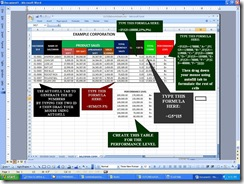# Using Single If and Multiple If of Microsoft Office Excel 2007IF function is a logical formula use to calculate or create conditions according to its given value in a worksheets

This worksheet I called Monthly commission of the salesman. All you have to do is to create this worksheets.Just like what I created or same as of the post picture I shown in this post. Just generate the salesman ID number by using the drag of your mouse to autofill the rest of the ID number on the preceding cells. It’s just a practice to perform our last post of the MS-Excel. Now input the name of the salesman and the monthly sales of its product. And add it up using the formula I presented in the post picture.

Now this is the problem of the worksheets: “The Company Example Corporation will give monthly commission of its salesman by 15 percent if they can sales up to 100000 or else 5 percent if they have lower sales of 100000 monthly.”

Using the single if function of the Microsoft Excel 2007 you will task to calculate the commission by percentage base of the total monthly sales of its of the salesman. And calculate the total commission of the salesman by multiplying its cells of Monthly Total Sales and commission by percentage. And using multiple if, you can calculate the performance level of its salesman base of its Total Monthly sales.

So, how to do this, just follow this simple steps:

1. Create the worksheet table same as the post picture I’ve shown in this post. You need to study first my last post in how to create a worksheets if you have no idea in creating a worksheet table.
2. Now generate the ID number same as the post picture I’ve shown
3. Now input the name of the salesman and monthly sales
4. Calculate the total monthly commission using the =sum function
5. Then find the commission by percentage using the formula I’ve shown in the post picture or use this: =IF(G5>100000,15%,5%)
6. And calculate the total commission using the formula I’ve shown in the post picture or use this: =G5*H5
7. Then calculate the performance level of the salesman base of the monthly sales using the multiple if. But before you put a formula you need to know first the range of the total sales or just follow this:
8.  PERFORMANCE LEVEL 200,000.00 170,000.00 A+ 169,000.00 130,000.00 B+ 149,000.00 119,000.00 C+ 118,000.00 108,000.00 D+ 107,000.00 105,000.00 E+ 104,000.00 70,000.00 F+ 69,000.00 80,000.00 G+
9. Then calculate the performance level using the formaula I’ve shown in the post picture or use this: =IF(G5>=170000,"A+",IF(G5>=130000,"B+",IF(G5>=119000,"C+",IF(G5>=108000,"D+",IF(G5>=105000,"E+",IF(G5>=70000,"F+",IF(G5>=80000,"F+")))))))
10. Then use the drag of your mouse using autofill tab to calculate the preceding cells.

If you have questions we love to hear your comments just type your questions in comment bar below or follow always this post for next update. “End”